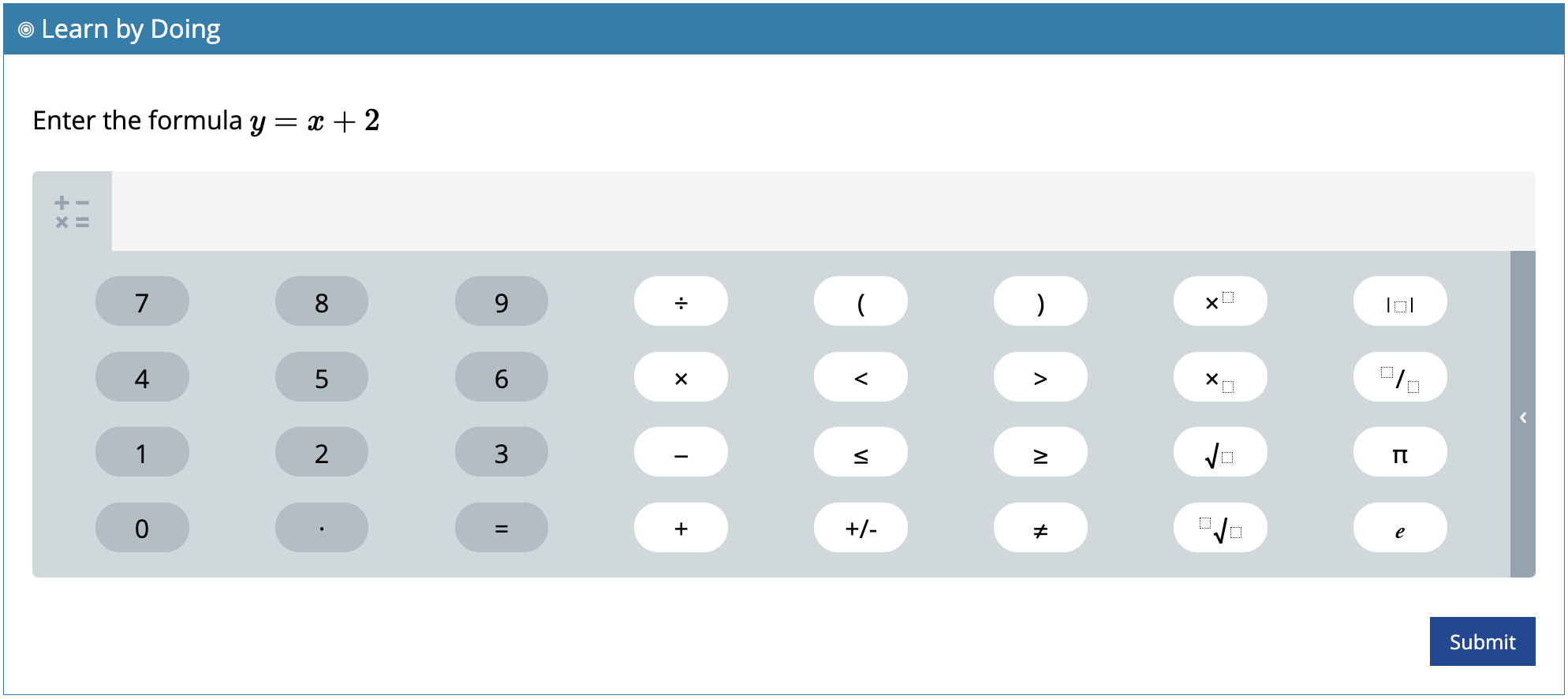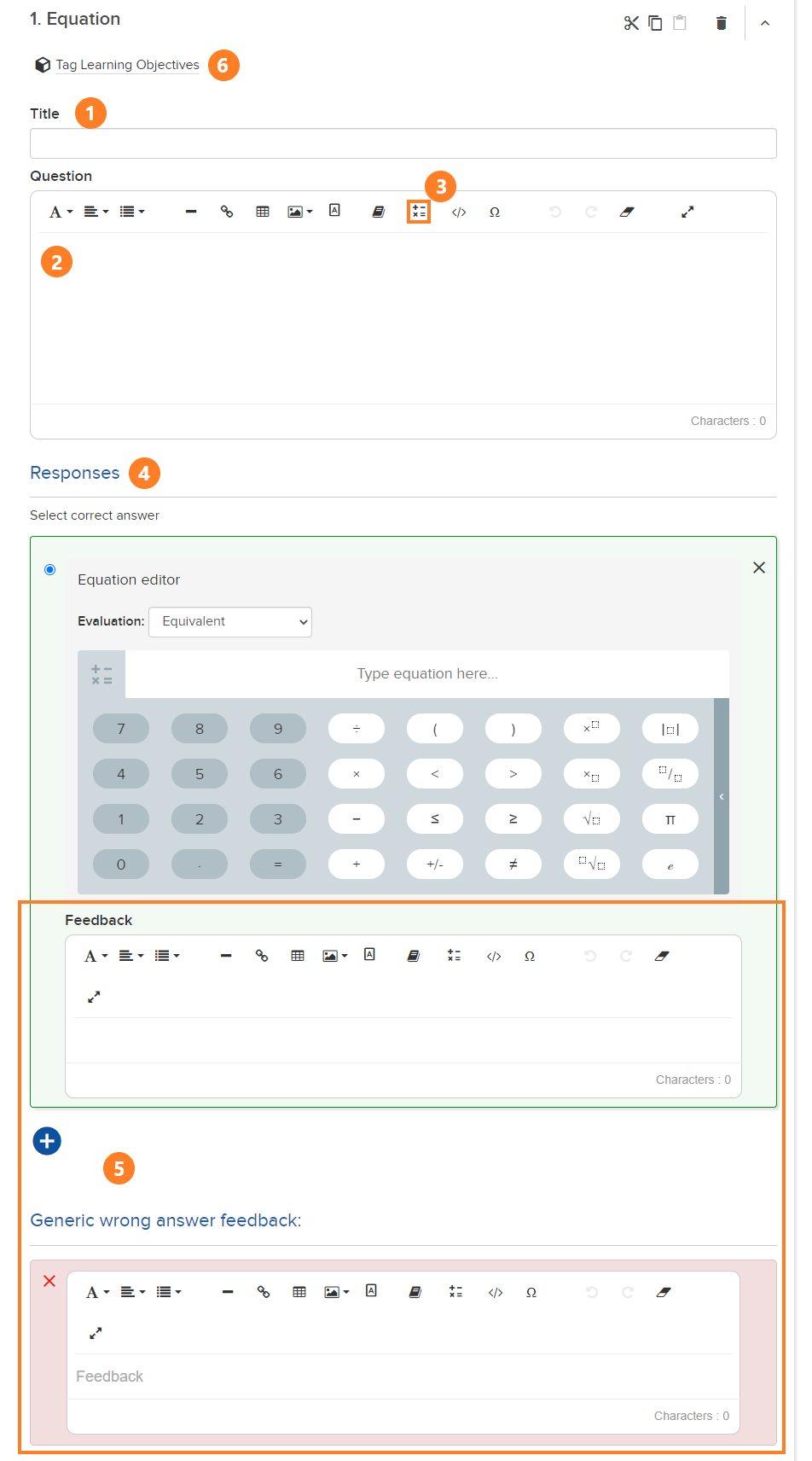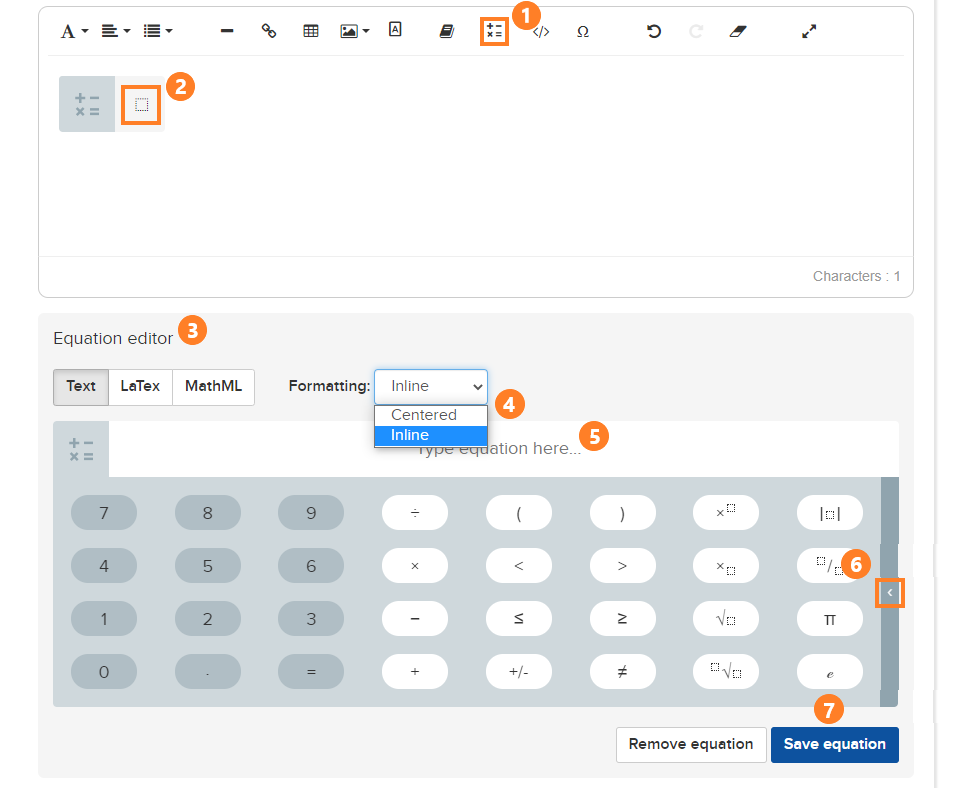# Equation

Equation questions are similar to numeric and text input question types. The Equation feature allows for equations in questions and requires students to input equations.

Sample:### Build an Equation Question

Click + New Assessment, choose the assignment type, name the assessment, click Add, click + Add Content or Question, click + Add Question, and then click Equation.

3. To include an equation as part of the question, click the equation icon indicated below and enter your equation in line. (For more information about the equation editor, see below.)

6. Tag question.### Equation Editor

The Equation Editor allows for creation of equations in text form, LaTex, or MathML. It provides access to keypad that allows for quick entry of commonly used math symbols and expressions. Using the Equation Editor assures that equations may be read with a screen reader.

1. Select the Equation tool from the toolbar.

2. Click in the equation box. The Equation Editor keypad opens.

3. Select Text, LaTex, or MathML for entering the equation.

4. Select Inline or Centered.

5. Type the equation in the space provided, or use the keypad to enter the equation.

6. You may expand the keypad by selecting the < icon (see below for expanded view).

7. Save or remove the equation.